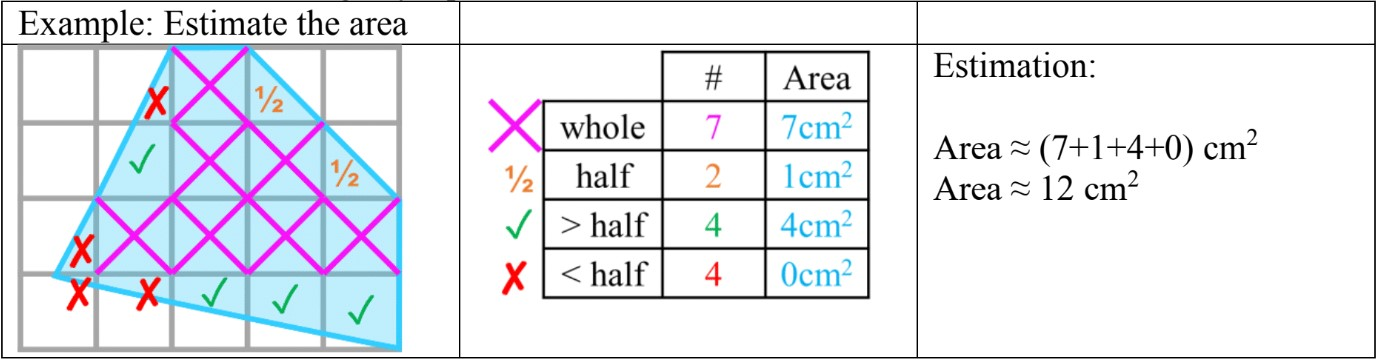# Calculating and Estimating Area and Perimeter#### All in One Place

Everything you need for JC, LC, and college level maths and science classes.#### Learn with Ease

We’ve mastered the national curriculum so that you can revise with confidence.#### Instant Help

24/7 access to the best tips, walkthroughs, and practice exercises available.

0/3
##### Intros
###### Lessons
1. Introduction to Calculating and Estimating Area and Perimeter:
2. Estimating area for triangles (using unit squares)
3. Estimating area for shaded in portions of unit squares
4. Example for estimating shaded area on a grid
0/8
##### Examples
###### Lessons
1. Comparing perimeter and areas of unit square rectangles
1. Fill in the table for each rectangle with proper units (cm)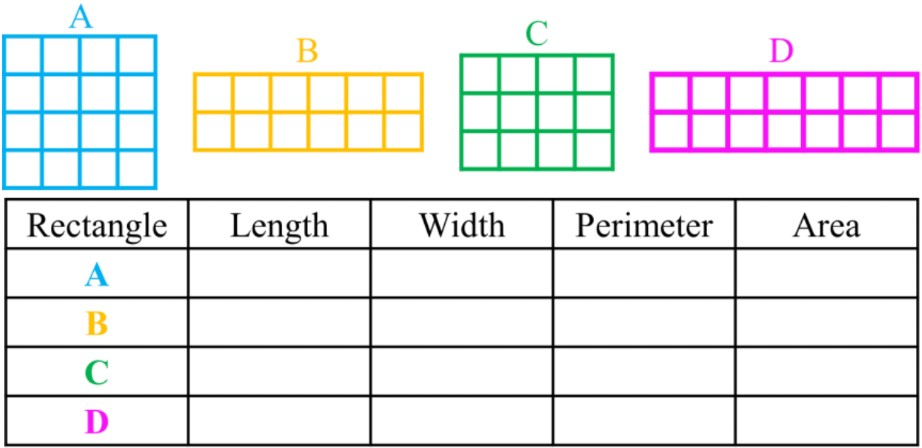2. Using the answers from part a), answer the following questions with the letter of each rectangle:
1. Which rectangle has the biggest perimeter? ____
2. Which rectangle has the biggest area? ____
3. Which rectangles have the same perimeter? ____
4. Which rectangles have the same area? ____
2. Estimating the area of a shaded figure using half unit squares
Estimate the area covered by the shaded figure.
Remember that: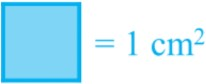and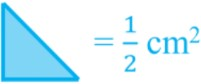1.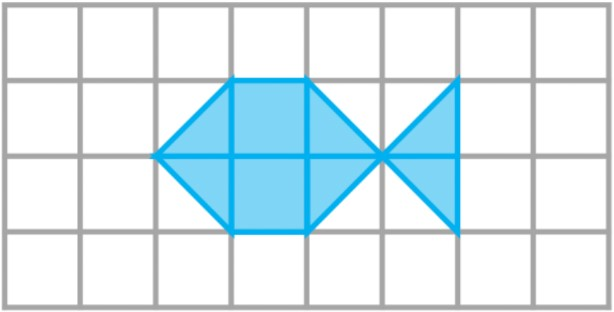2.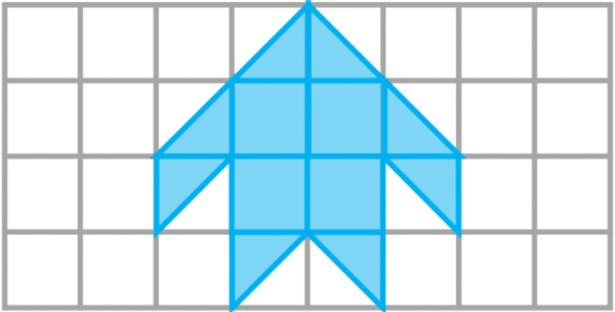3. Estimating the area of a shaded figure using halves of unit square doubles
Estimate the area covered by the shaded figure.
Remember that:and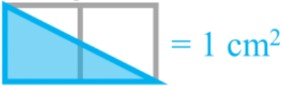1.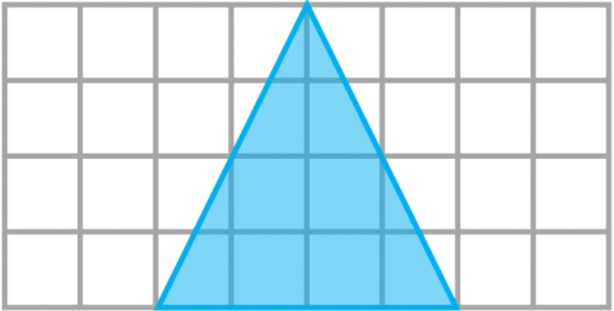2.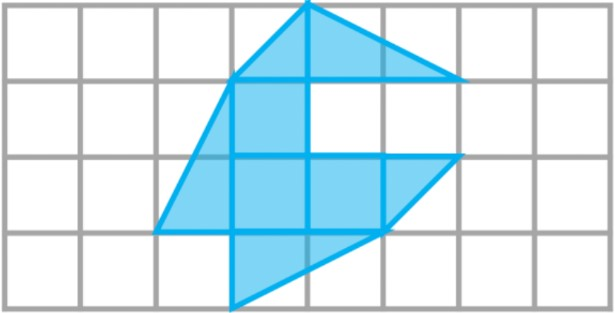4. Estimating the area of a shaded figure with different proportions of unit squares
Approximate the area of the shape by filling out the table.
1. How many of each type of square is shaded in?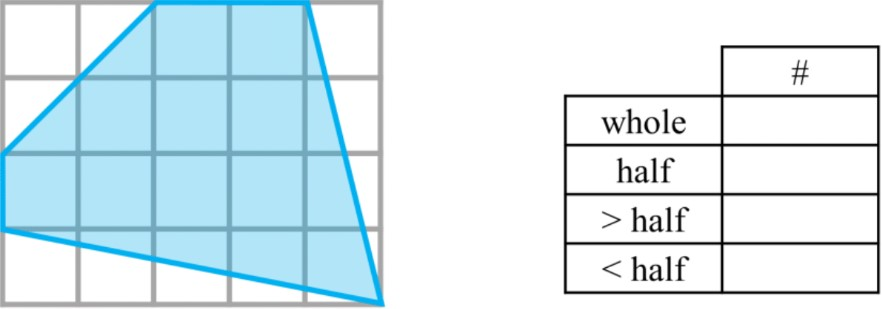2. What is the approximate area if each square represents 1m2?
The values of each type of square shaded in are listed:
1. whole = 1
2. half =1/2
3. $>$ half = 1
4. $<$ half = 0
###### Free to Join!
StudyPug is a learning help platform covering math and science from grade 4 all the way to second year university. Our video tutorials, unlimited practice problems, and step-by-step explanations provide you or your child with all the help you need to master concepts. On top of that, it's fun - with achievements, customizable avatars, and awards to keep you motivated.
• #### Easily See Your ProgressWe track the progress you've made on a topic so you know what you've done. From the course view you can easily see what topics have what and the progress you've made on them. Fill the rings to completely master that section or mouse over the icon to see more details.
• #### Make Use of Our Learning Aids###### Practice Accuracy

Get quick access to the topic you're currently learning.

See how well your practice sessions are going over time.

Stay on track with our daily recommendations.

• #### Earn Achievements as You LearnMake the most of your time as you use StudyPug to help you achieve your goals. Earn fun little badges the more you watch, practice, and use our service.
• #### Create and Customize Your AvatarPlay with our fun little avatar builder to create and customize your own avatar on StudyPug. Choose your face, eye colour, hair colour and style, and background. Unlock more options the more you use StudyPug.
###### Topic Notes

In this lesson, we will learn:

• How to count areas with squares and/or triangles (unit squares and half unit squares)
• How to estimate areas of shapes on an area grid

Notes:

• Review: finding perimeter and area for rectangular figures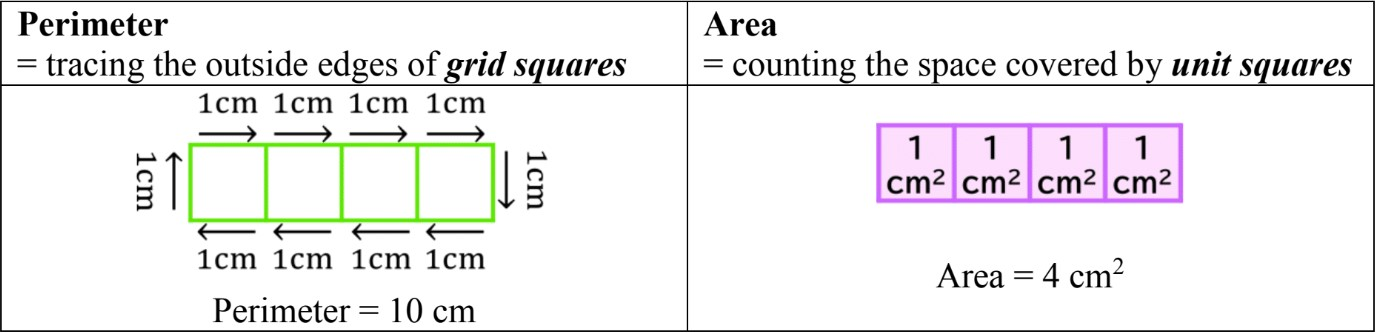• How do you find the area of shapes that are not made of squares?
• If you cut a square diagonally, you will get a triangle (half a square is a triangle)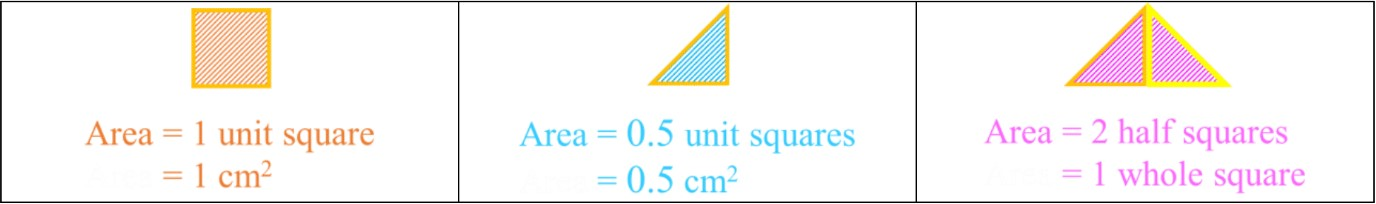• If you cut 2 squares diagonally (2 cm2), you will get half the area (1 cm2)
• There are other variations of getting half of a square (1/2 cm2 or 0.5 cm2)
• There will also be cases where you won't know the exact amount shaded in one square, but you can say whether it is more or less than half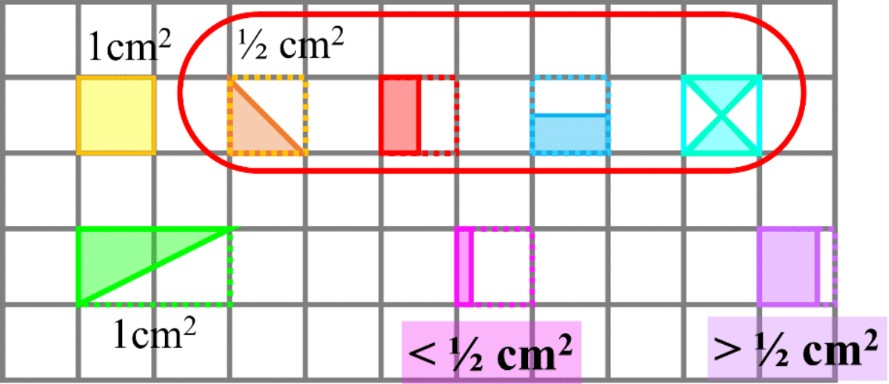• To estimate areas of a shape on a grid, we can get close to the actual area by:
• Counting all the whole unit squares (fully shaded in or covered by the shape)
• Counting all the half unit squares (most commonly the diagonal triangle)
• Rounding any squares shaded more than half up to $\approx$ 1 cm2
• Rounding any squares shaded less than half down to $\approx$ 0 cm2